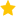See the live dashboard for CensusAtSchool 2023

Johanna McHardy (Mt Albert Grammar School) presented a variety of structured problems and solutions that help students understand and solve probability problems. The emphasis was on Venn diagrams, conditional probability and using tables and graphs.

Probability Venn Diagrams Word Word doc

Probability Venn Diagrams Power point

Revision Conditional Probability Word doc

Revision Conditional Probability Power point

• Event: 2014 Statistics Teachers' Day
• Keywords: Conditional probability, Venn Diagrams
• (Last updated: 15/05/18. Added: 12/12/14)
• ## Rate this resource:(1 ratings)